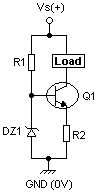# Zener diode - excuse me?

I've been playing, trying to create a zener driver current source as described in "The Art of Electronics" (Chapter 2.06 "Transistor Current Sources", 2nd Ed) (thinking towards an LED driver for a static, battery driven string) The circuit is as follows:Now, for testing, I've disconnected the base of Q1 so that I can check V(R1) and V(DZ1) to check that they stay in bounds that I'm expecting. R1=45k and DZ1 V(fwd)=3.9v

Vs = 3.40 V(DZ1) = 1.84 V(R1) = 1.56
Vs = 6.43 V(DZ1) = 2.087 V(R1) = 4.34
Vs = 9.44 V(DZ1) = 2.221 V(R1) = 7.22
Vs = 12.66 V(DZ1) = 2.321 V(R1) = 10.33

As I'm repeating these experiments, I realise that my R1 may be a little high to get a good enough holding current, so I'm repeating with R1=6k8 (which should give me an I(DZ1) of between 300 uA and 1.2mA) (The example in AoE is 10k)

Vs = 3.39 V(DZ1) = 2.288 V(R1) = 1.116
Vs = 6.43 V(DZ1) = 2.658 V(R1) = 3.77
Vs = 9.44 V(DZ1) = 2.848 V(R1) = 6.59
Vs = 12.69 V(DZ1) = 2.985 V(R1) = 9.67

The I(DZ1) @ Vs=12.69 is measured at 1.42mA - which works out about 5mW (1% of rated power). You can get LEDs that will light at 2mA!

So here's my question - why is this not asymptoting to 3.9V? Do I really needs a higher I(DZ1) to get this thing to hold its rated zener voltage?

One closing set of values ...

R1 = 1k
Vs = 6.43
I(DZ1) = 3.13mA
V(DZ1) = 3.28
V(R1) = 3.144

Over 3mA and it's still not there yet.

Pol.

Yup, that's normalWhat part number are you using for the zener diode? Some zener diodes have their rated voltage specified at 20mA!

--
The Ruggeduino: compatible with Arduino UNO, 24V operation, all I/O's fused and protected

It's a 1N5228B http://www.farnell.com/datasheets/89267.pdf. Now you've raised the idea of 20mA being normal, that datasheet makes a bit more sense. At no point does it say the column with 20mA in is the minimum/advised/typical value - useful.

The example in AoE has a current of 940uA. (15V supply, 10k R1 and 5.6V zener)

Thanks for the pointer.

At no point does it say the column with 20mA in is the minimum/advised/typical value - useful.

It does but in a very roundabout way. Notice that Vz(V)@Iz column lists 3.9V as the typical value, while the next column over lists Zz(Ohms)@Iz(mA) and that is where Iz is revealed to be 20mA. "Better" datasheets will actually show Iz vs. Vz values for some typical cases in the form of graphs and you will see that as the Vz voltages get lower, the dependence on current gets higher.

--
BeatVox: inexpensive sound shield for Arduino, 3.5mm stereo output, 512Kbyte FLASH

IIRC low-voltage 'zeners' are not true zener diodes at all and don't work by avalanche breakdown (thus the 'soft' knee in the curve). Higher voltage devices probably have better behaviour. In that datasheet you can see the change from positive to negative temp-co as you move to lower voltage devices - this is where the diodes change from zener to the other sort... (also the dynamic resistance value changes sharply at that point). More about this in Art of Electronics I believe.

That rings a bell from college 45 years ago MarkI had forgotten about the low voltage zeners not actually being zeners, more like diodes in series isnt it?

and I seem to recall the "best" voltage for thermal drift was 4v7 ?

A better solution is to replace the zener with a NPN transistor. Any TO-92 will do. Emitter to ground. Collector to base of existing transistor. Base to emitter of existing transistor.
Size R2 for 0.6 volts at whatever current you require.
Advantage of this, for driving Led's, is a negative temperature co-efficient. As Q2 gets hotter, it's Vbe drops, decreasing the current with it.

Amanda

I have also used a single transistor, with a low value resistor from its emitter to ground, and the base having a resistor to + and an LED to ground, that's also a total of 4 components, and I read that the temperature coefficient of the transistor and LED cancelled out.

I passed about 5 mA through the LED and it worked very well.

Boffin1:
That rings a bell from college 45 years ago MarkI had forgotten about the low voltage zeners not actually being zeners, more like diodes in series isnt it?

and I seem to recall the "best" voltage for thermal drift was 4v7 ?

A little investigation reveals that the Zener effect is the low voltage one (a tunnelling phenomenon) and avalanche breakdown is the higher voltage mechanism. Around 6V both mechanisms can be present together and the temperature dependence of voltage is least...